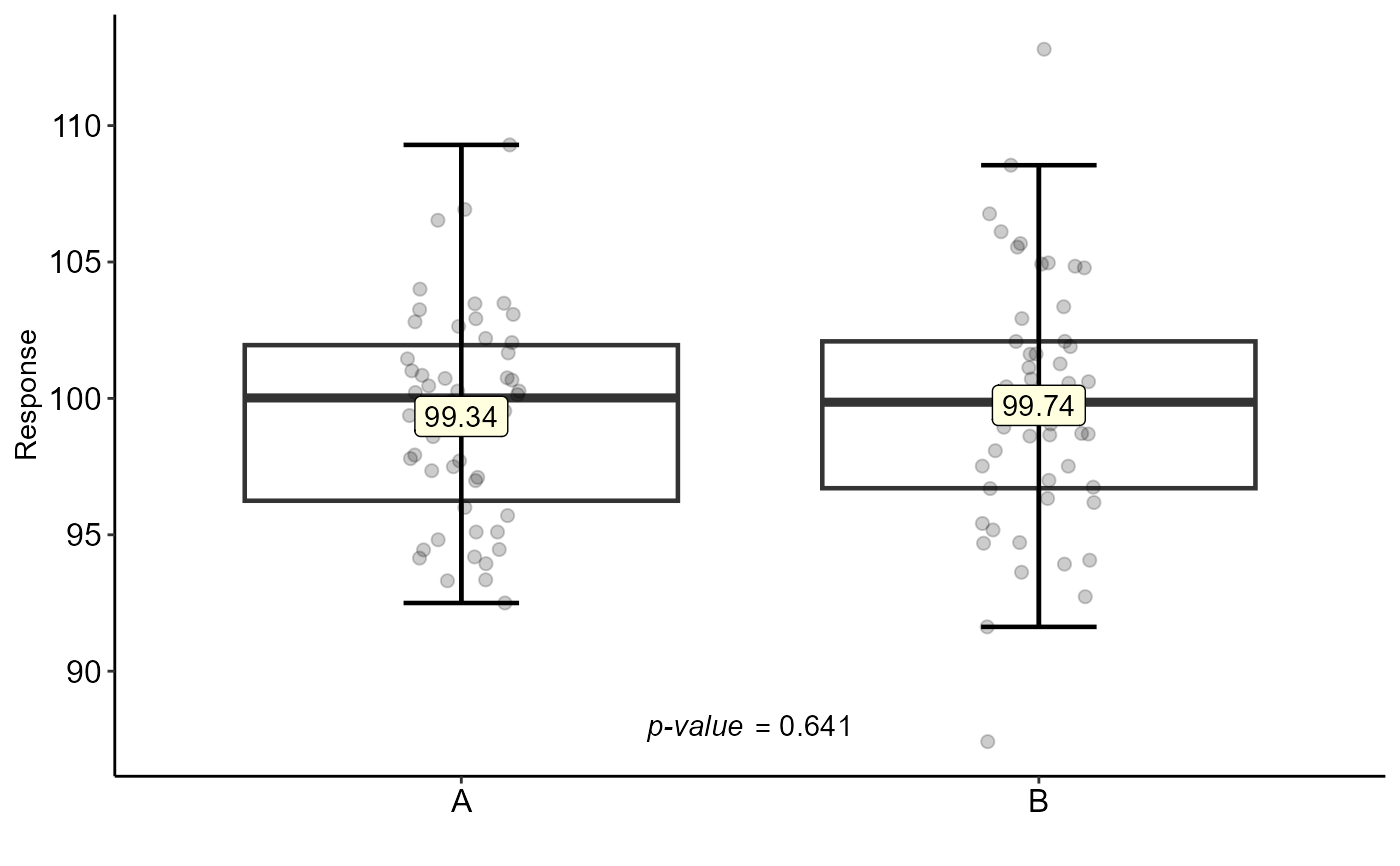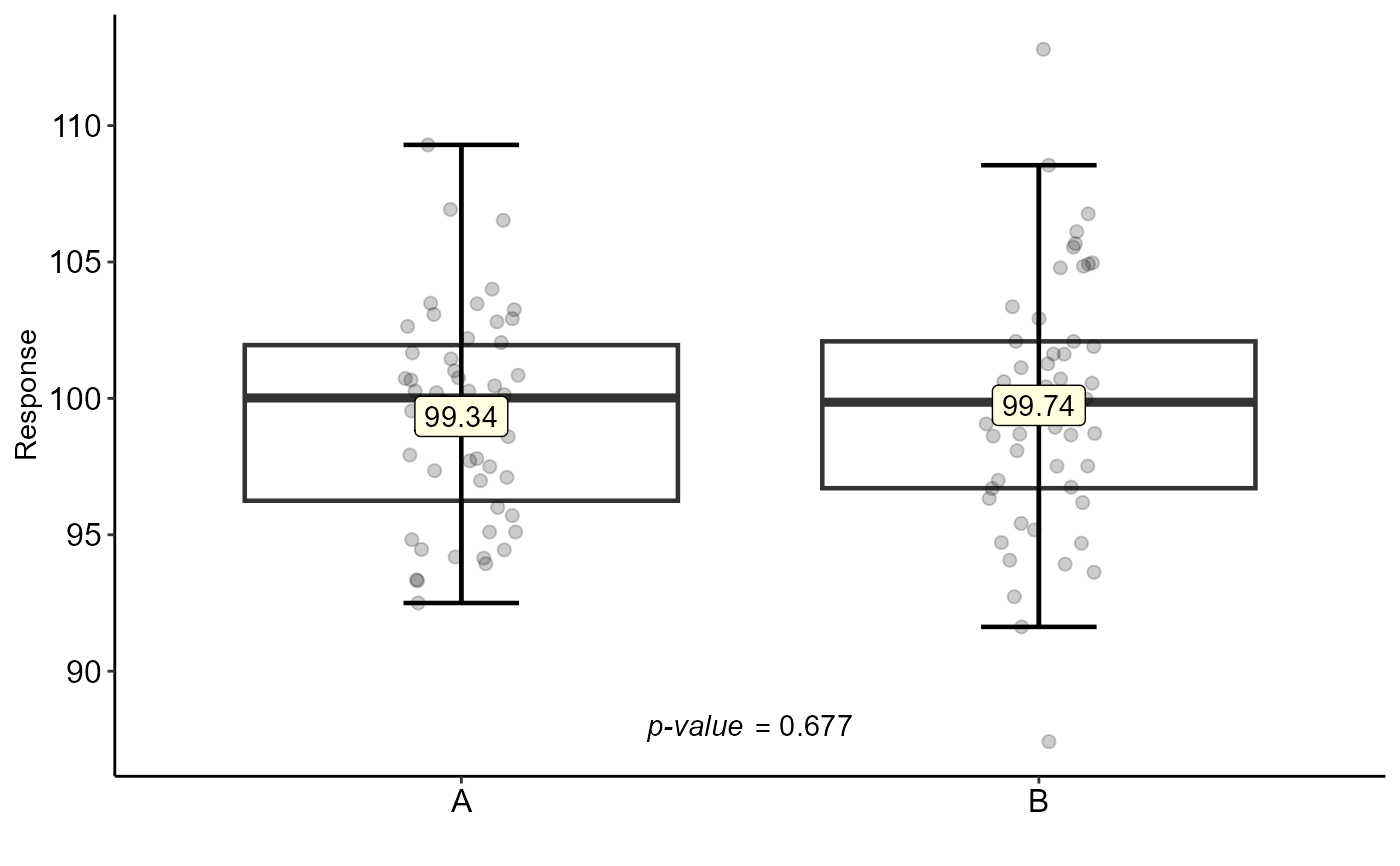Test for two samples (paired and unpaired t test, paired and unpaired Wilcoxon test)

test_two(
trat,
resp,
paired = FALSE,
correct = TRUE,
test = "t",
alternative = c("two.sided", "less", "greater"),
conf.level = 0.95,
theme = theme_classic(),
ylab = "Response",
xlab = "",
var.equal = FALSE,
pointsize = 2,
yposition.p = NA,
xposition.p = NA,
fill = "white"
)

## Arguments

trat

Categorical vector with the two treatments

resp

Numeric vector with the response

paired

A logical indicating whether you want a paired t-test.

correct

A logical indicating whether to apply continuity correction in the normal approximation for the p-value.

test

Test used (t for test t or w for Wilcoxon test)

alternative

A character string specifying the alternative hypothesis, must be one of "two.sided" (default), "greater" or "less". You can specify just the initial letter.

conf.level

Confidence level of the interval.

theme

ggplot2 theme (default is theme_classic())

ylab

Variable response name (Accepts the expression() function)

xlab

Treatments name (Accepts the expression() function)

var.equal

A logical variable indicating whether to treat the two variances as being equal. If TRUE then the pooled variance is used to estimate the variance otherwise the Welch (or Satterthwaite) approximation to the degrees of freedom is used.

pointsize

Point size

yposition.p

Position p-value in y

xposition.p

Position p-value in x

fill

fill box

## Value

Returns the test for two samples (paired or unpaired t test, paired or unpaired Wilcoxon test)

## Details

Alternative = "greater" is the alternative that x has a larger mean than y. For the one-sample case: that the mean is positive.

If paired is TRUE then both x and y must be specified and they must be the same length. Missing values are silently removed (in pairs if paired is TRUE). If var.equal is TRUE then the pooled estimate of the variance is used. By default, if var.equal is FALSE then the variance is estimated separately for both groups and the Welch modification to the degrees of freedom is used.

If the input data are effectively constant (compared to the larger of the two means) an error is generated.

## Author

Gabriel Danilo Shimizu, shimizu@uel.br

Leandro Simoes Azeredo Goncalves

Rodrigo Yudi Palhaci Marubayashi

## Examples

resp=rnorm(100,100,5)
trat=rep(c("A","B"),e=50)
test_two(trat,resp)
#>
#> 	Welch Two Sample t-test
#>
#> data:  resp by trat
#> t = -0.46798, df = 94.135, p-value = 0.6409
#> alternative hypothesis: true difference in means between group A and group B is not equal to 0
#> 95 percent confidence interval:
#>  -2.115030  1.308182
#> sample estimates:
#> mean in group A mean in group B
#>        99.34043        99.74385
#>test_two(trat,resp,paired = TRUE)
#>
#> 	Paired t-test
#>
#> data:  resp by trat
#> t = -0.41949, df = 49, p-value = 0.6767
#> alternative hypothesis: true difference in means is not equal to 0
#> 95 percent confidence interval:
#>  -2.336029  1.529181
#> sample estimates:
#> mean of the differences
#>              -0.4034238
#>